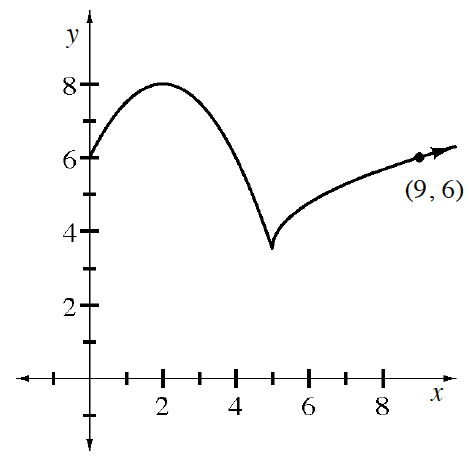### Home > APCALC > Chapter 1 > Lesson 1.3.2 > Problem1-113

1-113.

Write a piecewise-defined function that will generate the graph at right.

Notice that there are two pieces that meet at $x = 5$. So the boundary will be $x = 5$.

Examine the first piece. What parent graph does it resemble? What transformation has been made? Write its equation.

Examine the second piece. What parent graph does it resemble? What transformation has been made? Write its equation.

Since the $y$-intercept and the point $(9,6)$ are given on the graph, you can check your work for steps 2 and 3. Here's how: Substitute $(0,6)$ to the first piece (does it work?) Substitute $(9,6)$ to the second piece (does it work?)

Assemble the piecewise function. Remember that the boundary is $x = 5$.

Use the eTool below to view the graphs.
Click the link at right for the full version of the eTool: Calc 1-113 HW eTool